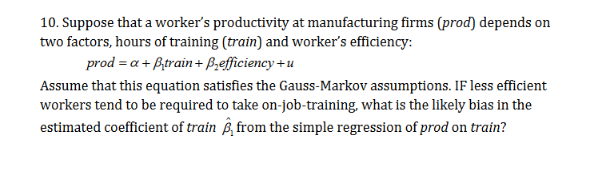# 10. Suppose that a worker's productivity at manufacturing firms (prod) depend:s ontwo factors, hours of training (train) and worker's efficiency:prod a+Btrain+ Prefficiency+uAssume that this equation satisfies the Gauss-Markov assumptions. IF less efficientworkers tend to be required to take on-job-training, what is the likely bias in theestimated coefficient of train £, from the simple regression of prod on train?

Question
8 viewshelp_outlineImage Transcriptionclose10. Suppose that a worker's productivity at manufacturing firms (prod) depend:s on two factors, hours of training (train) and worker's efficiency: prod a+Btrain+ Prefficiency+u Assume that this equation satisfies the Gauss-Markov assumptions. IF less efficient workers tend to be required to take on-job-training, what is the likely bias in the estimated coefficient of train £, from the simple regression of prod on train? fullscreen
check_circle

Step 1

According to the provided data, the equation satisfies the Gauss-Markov assumptions. If less efficient workers tend to be requi...

### Want to see the full answer?

See Solution

#### Want to see this answer and more?

Solutions are written by subject experts who are available 24/7. Questions are typically answered within 1 hour.*

See Solution
*Response times may vary by subject and question.
Tagged in

### Statistics